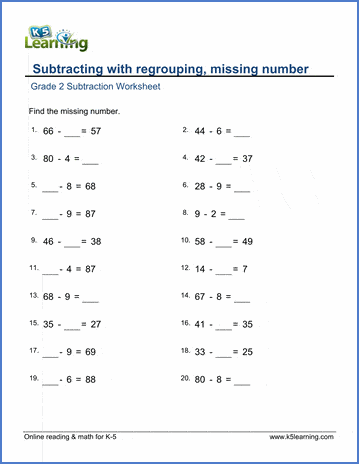# Subtracting from a 2-digit number; missing number

## Worksheets: Subtracting a 1-digit number from a 2-digit number

Below are six versions of our grade 2 math worksheet on subtracting a 1-digit number from a 2-digit number; regrouping (or borrowing) is required for most questions.  Students must find the missing number in the equation.  These worksheets are pdf files.## More subtraction worksheets

Explore all of our subtraction worksheets, from subtracting by counting objects to subtracting large numbers in columns.

## What is K5?

K5 Learning offers reading and math worksheets, workbooks and an online reading and math program for kids in kindergarten to grade 5.  We help your children build good study habits and excel in school.